For the propagation equation, an analytical solution typically can not be obtained, since the nonlinear Schroedinger equation used as the propagation equation is a nonlinear partial differential equation. Therefore, a numerical analysis is employed for understandings of nonlinear optical effects yielded in an optical fiber. In the following paragraphs, we focus on split step Fourier method as a calculation method and explain a method to analyze behaviours of pulse in an optical fiber. Because  the split step Fourier method employs an algorithm of fast Fourier transform (FFT), the analysis speed is faster than most of other analytical methods.

In order to explain the split step Fourier method, we use the Nonlinear Schroedinger equation. First of all, we deform the Nonlinear Schroedinger equation into the following expression,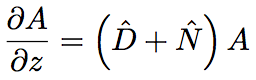where D^ is a differential operator representing dispersion and absorption of linear medium, and N^ is a nonlinear operator representing an influence of fiber nonlinearity to pulse propagation. These operators are given as follows.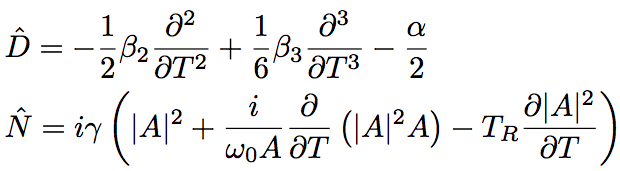in general case, the dispersion and the nonlinearity appears at the same time along the fiber axial direction. In the split step Fourier method, assuming that the dispersion effect and the nonlinear optical effect appears separately when light travels for a short distance, h, in an optical fiber, an approximated solution is calculated. More concretely, the light propagation from z to z+h is considered as the following three steps. Figure shows the schematic of three steps.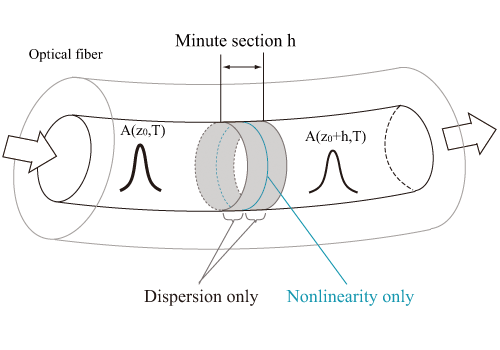Fig. Split step Fourier method

➀Assume that only the dispersion effect appears in the first region of the tiny interval, h, and consider the light propagation for N^ = 0. ➁Assume that only the nonlinear optical effect appears at the center of tiny interval and D^ = 0, and operate the nonlinear optical effect corresponding to the whole interval, h. ➂Consider that only the dispersion effect works at the remaining interval of h/2. These steps are expressed by the following equation.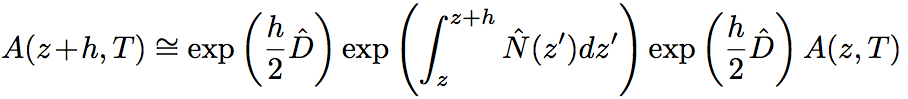At first, assuming that a pulse propagates only by 2/h in the tiny interval at the beginning, the exponential operator, exp(jD^/2), is operated in the Fourier space as shown below.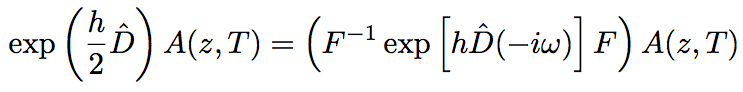F is an operator for Fourier transform, and D~(-iω) is an alternative of the differential operator (∂/∂T is alternated by -iω). ω is a frequency in the Fourier space. Since ∂/∂T is just a figure in the Fourier space, the equation above can be directly calculated. By using an FFT algorithm, a numerical calculation of the equation above can be performed rapidly.

Second, the nonlinear optical effect corresponding to the whole interval is operated at the center of tiny space, h. As shown in the equation describing A(z+h, T), the integral over the whole interval is operated. By utilizing a pedestal approximation, a precise approximation solution is obtained as shown below.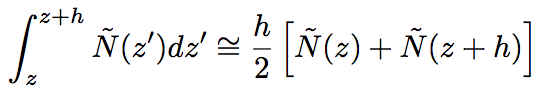However, since N~(z+h) is not obtained at the center, z+h/2, it is difficult to utilize the equation above without any modification. For the integral calculation, an iteration is used with setting a initial of N~(z+h) as N~(z). A(z+h,T) is derived, then a new value of N~(z+h) is calculated. Although the iteration calculation requires a long time, the calculation method based on this algorithm gives a result with a high accuracy. By using a larger interval step, h, the total calculation time becomes relatively shorten. In practice, twice iteration calculations are quite enough. Finally, assuming the propagation in the remaining h/2 region with considering only the dispersion, A(z+h,T) is derived.

In utilizing the split step Fourier method, we have to pay a lot of attentions to total time range, temporal resolution, propagating step range, and so on. Especially, the total time range has to be sufficiently large with considering a time delay among each frequency component through the propagation. This is because a component reaching at the boundary of total time range is automatically input at the opposite side, resulting an instability of numerical calculation. We also have to be careful of the relationship between the total time range and the resolution of spectrum domain (Fourier space).

The temporal resolution has to be sufficiently better than a duration of propagating pulse. The optimal value of propagating step range depends on a complexity of issue. In case a large nonlinear optical effect is operated, a small propagating step range is required. Thus, by setting appropriate parameters, the split step Fourier method becomes the powerful analytical tool.